# Electronics and Communication Engineering - Microwave Communication

Exercise : Microwave Communication - Section 6
36.
As compared to their values in free space
velocity of electric wave in dielectric is smaller but wave length of electric wave is more
both velocity and wave length of electric wave in dielectric are higher
both velocity and wave length of electric wave in dielectric are smaller
wave length of electric wave is less but velocity is more in dielectric
Explanation:
No answer description is available. Let's discuss.

37.
A 3 port circulator is in the given figure. Its scattering matrix is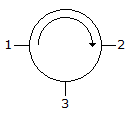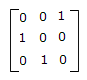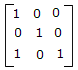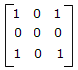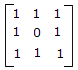Explanation:
No answer description is available. Let's discuss.

38.
Characteristic impedance of a coaxial cable is inversely proportional to r.
True
False
Explanation:
No answer description is available. Let's discuss.

39.
A rectangular waveguide has a discontinuity in the form of reduced narrow dimension. In the equivalent circuit this discontinuity can be represented by
a shunt capacitance at the discontinuity
a shunt inductance at the discontinuity
a shunt resistance at the discontinuity
none of the above